# Average Questions for Competitive Exams

Average Questions of Quantitative Aptitude ( Maths) for Competitive Exams. Topic wise Average question and answer with solution from the previous year exam question paper of SSC CGL, CPO, CHSL, RRB, bank. Practice Set is useful for upcoming NRA CET, UPSSSC PET and other Govt jobs examinations.

### Basic Average Questions

Q1. Average runs scored by 11 players of a cricket team is 23 runs. If the first player scored 113 runs. Find the average runs of the remaining players.

1) 8 Runs
2) 12 Runs
3) 14 Runs
4) 27 Runs

Ans : 3) 14 Runs
Total Run scored = 11×23 =253
Run of First player = 113
Run by rest players = 253-113 = 140
Average = 140/10 = 14

Q2. The average weight of 15 oarsmen in a boat is increased by 1.6 kg when one of the crew, who weighs 42 kg, is replaced by a new man. Find the weight of the new man (in kg).

1) 67
2) 65
3) 66
4) 43

Ans : 3) 66
Weight of new oarsman
= (42 + 15 × 1.6) kg
= (42 + 24) kg = 66 kg

Q3. The average marks of 50 students in a class is 72. The average marks of boys and girls in that subject are 70 and 75 respectively. The number of boys in the class is

1) 20
2) 35
3) 25
4) 30

Ans : 4) 30
Let number of Boys = x
Number of Girls = 50 -x
x × 70 + (50 − x) × 75 = 50 × 72
⇒ 70x + 3750 − 75x = 3600
⇒ 3750 − 5x = 3600
⇒ 5x = 3750 − 3600 = 150
⇒ x=30

Q4. The average weight of 8 persons increases by 2.5 kg when a new person comes in place of one of them weighing 65 kg. The weight of the new person is

1) 84 Kg
2) 85 Kg
3) 76 Kg
4) 76.5 Kg

Ans : 2) 85 Kg
Weight of new person
= (65 + 8 × 2.5) kg
= (65 + 20) kg = 85 kg

Q5. Six friends have an average height of 167 cm. A boy with height 162 cm leaves the group. Find the new average height.

1) 168 cm
2) 166 cm
3) 169 cm
4) 167 cm

Ans : 1) 168 cm
Total height of 5 friends = (6 × 167 − 162) cm= (1002 − 162) cm = 840 cm
Required average = 840/5 = 168 cm

Q6. The average of 1, 3, 5, 7, 9, 11, ………… to 25 terms is

1) 125
2) 25
3) 625
4) 50

Ans : 2) 25
The average of first n odd natural number is n

Q7. The average of some natural numbers is 15. If 30 is added to first number and 5 is subtracted from the last number the average becomes 17.5 then the number of natural number is

1) 15
2) 30
3) 20
4) 10

Ans : 4) 10
Let there are x natural numbers
Sum = 15 x
15x + 30 − 5 = x × 17.5
⇒ 17.5x − 15x = 25
⇒ 2.5x = 25
⇒ x = 10

Q8. There are 100 students in 3 sections A, B and C of a class. The average marks of all the 3 sections were 84. The average of B and C was 87.5 and the average marks of A is 70. The number of students in Section A was

1) 30
2) 35
3) 20
4) 25

Ans : 3) 20
If the number of students in section A be x.
Therefore, the number of students in sections B and C will be = (100 − x )
Now we have
x × 70 + (100 − x) × 87.5 = 84 × 100
⇒ 70x + 87.5 × 100 − 87.5 x = 8400
⇒ 8750 − 17.5x = 8400
⇒ 17.5x = 8750 − 8400 = 350
⇒ x = 20

Q9. The average of all the numbers between 6 and 50 which are divisible by 5 is

1) 27.5
2) 30
3) 28.5
4) 22

Ans : 1) 27.5
Numbers are: 10, 15, 20, 25, 30, 35, 40, 45
Sum of numbers = 220
Total number = 8
Average = 220/8 = 27.5

Q10. The average of 20 numbers is 15 and the average of first five is 12. The average of the rest is

1) 16
2) 15
3) 14
4) 13

Ans : 1) 16
Let the average of remaining 15 numbers are x
20 × 15 = 5 × 12 + 15x
⇒ 15x = 300 − 60 = 240
⇒ x = 240/15=16

Q11. The average of 8 numbers is 27. If each of the numbers is multiplied by 8, find the average of new set of numbers.

1) 1128
2) 938
3) 316
4) 216

Ans : 4) 216
Average gets multiplied by 8.
Required average = 8 × 27 = 216

Q.12: 4 boys and 3 girls spent 120 on the average, of which the boys spent 150 on the average. Then the average amount spent by the girls is :
a) ₹ 80
b) ₹ 60
c) ₹ 90
d) ₹ 100

Ans : a) ₹ 80
Total expenditure = 120 × 7 = 840
Total expenditure of 4 boys = 150 × 4 =600
Total expenditure of 3 girls = 840 − 600 = 240
Average expenditure of Girls = 240/3 =80

Q.13: The mean of 9 observations is 16. One more observation is included and the new mean becomes 17. The 10th observation is :
a) 9
b) 16
c) 26
d) 30

Ans : c) 26
Tenth observation = Mean of ten observations – Mean of nine observations
= 10 × 17 − 16 × 9
= 170 − 144 = 26

Q.14: 12 kg of rice costing ₹ 30 per kg is mixed with 8 kg of rice costing ₹ 40 per kg. The average per kg price of mixed rice is :
a) ₹ 38
b) ₹ 37
c) ₹ 35
d) ₹ 34

Ans : d) ₹ 34
= (12 × 30 + 8 × 40) =680
Average per kg price = 680 /20 = 34

Q.15: The average of 30 results is 20 and the average of other 20 results is 30. What is the average of all the results?
a) 24
b) 48
c) 25
d) 50

Ans : a) 24
Total = 20×30 + 20×30 = 1200
Average = 1200/50 = 24

Q.16: The average of the marks obtained in an examination by 8 students was 51 and by 9 other students were 68. The average marks of all 17 students were :
a) 59
b) 59.5
c) 60
d) 60.5

Ans : c) 60
Sum of total marks = 8×51 + 9×68 = 408 + 612 = 1020
Total Students = 8+9 =17
Average = 1020/17 = 60

### To Find nth Number When Average of First ‘p’ and Last ‘q’ Numbers are Given

Q.17: Out of four numbers the average of the first three is 16 and that of the last three is 15. If the last number is 20 then the first number is ?
a) 23
b) 25
c) 28
d) 21

Ans : a) 23
Let number are a, b, c, and 20
a + b + c = 16 × 3 = 48 -> (1)
b + c + 20 = 15 × 3 = 45 ->(2)
(1) – (2) = a – 20 = 48 -45 = 3
=> a = 23

Q.18: The average of 13 results is 70. The average of first seven is 65 and that of the last seven is 75, the seventh result is :
a) 67
b) 70
c) 68
d) 70.5

Ans : b) 70
Seventh Result is = (65 × 7) + (7 × 75) − (13 × 70)
= 455 + 525 – 910
= 980 – 910 = 70

Q.19: The average of 12 numbers is 15 and the average of the first two is 14. What is the average of the rest?
a) 15
b) 15.2
c) 14
d) 14.5

Ans : b) 15.2
Sum of remaining 10 numbers = (12 × 15) − (2 × 14) = 180 − 28 = 152
Average = 152/10 = 15.2

Q.20: Out of four numbers, the average of the first three is 18 and that of the last three is 16. If the last number is 19, the first is :
a) 19
b) 18
c) 20
d) 25

Ans : d) 25
a + b + c = 18 × 3 = 54 (1)
b+ c + 19 = 16 × 3 = 48 (2)
(1) – (2) = a-19 =6 => a = 6+19 = 25

Q.21: The average of three numbers is 135. The largest number is 195 and the difference between the other two is 20. The smallest number is :
a) 65
b) 105
c) 95
d) 115

Ans : c) 95
Let Smallest number is a, other number is a+20
a + (a +20) + 195 = 135 x3 = 405
=> 2a = 405 -215 = 190, => a = 95

Q.22: The average of eight successive numbers is 6.5. The average of the smallest and the greatest numbers among them will be :
a) 4
b) 6.5
c) 7.5
d) 9

Ans : b) 6.5
x +(x+1)+(x+2)+(x+3)+(x+4)+(x+5)+(x+6)+(x+7) = 6.5 x8
=> 8x +28 =52, => x = 3
smallest and the greatest numbers are = 3 and 10
Average = 13/2 = 6.5

### Average of Consecutive Even, Odd and Prime Numbers

Q.23: The average of five consecutive positive integers is n. If the next two integers are also included, then the average of all these integers will :
a) Increased by 1.5
b) Increased by 1
c) Remain the same
d) Increase by 2

Ans : b) Increased by 1
Average of five consecutive numbers =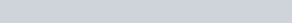$\frac{ a+(a+1)+(a+2)+(a+3)+ (a+4)} { 5} =\frac{(5a+10)}{5} = a+2$
New average after adding two numbers =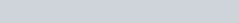$\frac{(5a+10) +(a+5)+(a+6)}{7} = \frac{7a+ 21}{7}= a+3$
Difference in average = (a+3)-(a+2) = 1

Q.24: The average of the first nine integral multiples of 3 is :
a) 12
b) 15
c) 18
d) 21

Ans : b) 15
Numbers are => 3(1+2+3+….+9)
Sum =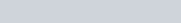$3(\frac {n(n+1)}{2}) = 3(\frac {9 \times 10}{2}) = 135$
Average = 135/9 = 15

Q.25: The average of odd numbers up to 100 is :
a) 49
b) 49.5
c) 50
d) 50.5

Ans : c) 50
Number of odd number up to 100 are 50
Therefore Average is 50

Q. 26: The average of nine consecutive odd numbers is 53. The least odd number is :
a) 22
b) 27
c) 35
d) 45

Ans : d) 45
Sum of nine consecutive odd numbers = a+(a+2)+(a+4)+(a+6)+(a+8)+(a+10)+(a+12)+(a+14) +(a+16)= 9 x53
=> 9a +72 = 477, => 9a = 405 => a = 45

### Average Monthly Income : Questions for Competitive Exams

Q.26: The average expenditure of a man for the first five months is 1200 and for the next seven months is ₹ 1300. If he saves ₹ 2900 in that year, his monthly average income is :
a) ₹ 1400
b ₹ 1500
c) ₹ 1600
d) ₹ 1700

Ans : b) ₹ 1500
Annual Expenditure = (5×1200 +7×1300) =15100
Annual Income = 15100 + 2900 = 18000
Average Monthly Income = 18000/12 = 1500

Q.27: The average salary of all the workers in a workshop is ₹ 8000. The average salary of 7 technicians is` ₹ 12,000 and the average salary of the rest is ₹ 6000. The total number of workers in the workshop is :
a) 20
b) 21
c) 22
d) 23

Ans : b) 21
Let total workers are A.
7 x 12000 + (A-7) x 6000 = 8000 A
=> 84000 + 6000 A – 42000 = 8000 A
=> 2000 A = 42000
=>A = 21

Average (Maths) Questions for Competitive Exams

### Twice, Thrice, One-Third, etc., of Numbers.

Q.28: Of the three numbers, the first is 4 times the second and 3 times the third. If the average of all the three numbers is 95, what is the third number?
a) 57
b) 76
c) 130
d) 60

Ans : d) 60
Third number A, First Number 3A, Second number = 3A/4
3A + 3A/4 + A = 3×95 = 285
=> 19 A = 285×4, => A = 60

Q. 29: Of the three numbers, the first number is twice the second and the second is thrice the third number. If the average of these 3 numbers is 20, then the sum of the largest and the smallest numbers is :
a) 24
b) 42
c) 54
d) 60

Ans b) 42
Third number – A, Second = 3A, First number 6A
6A +3A+A = 3×20 =60,
=> 10A = 60 => A = 6
Sum of the largest and the smallest numbers is = 6A+A = 7A = 7×6 = 42

Q. 30: Of the three numbers, the first is twice the second and the second is twice the third. The average of three numbers is 21. The smallest of the three numbers is :
a) 6
b) 9
c) 12
d) 18

Ans : b) 9
Third number =A, Second 2A, First 4A
A+2A+4A = 21 x 3 = 63
7A = 63 => A =9

Average Questions with solutions for Competitive Exams

### Correct Average for mistake done earlier

Q.31: The average marks of a class of 35 children is 35. The marks of one of the students, who got 35, was incorrectly entered as 65. What is the correct average of the class?
a) 34.14
b) 28.20
c) 42.21
d) 38.14

Ans : a) 34.14
Total incorrect Marks = 35×35 = 1225
Incorrectly added : 65-35 = 30
Total Correct marks = 1225-30 =1195
Correct Average 1195/35 =34.14

Q.32: The average marks secured by 36 students were 52. But it was discovered that an item 64 was misread as 46. What is the correct mean of marks?
a) 52.0
b) 52.5
c) 53.0
d) 53.5

Ans : b) 52.5
Difference of correct and incorrect marks = 64 − 46 = 18
∴ Correct mean = 52 + 18/36 = 52.5

### Cricket Based : Average Questions for Competitive Exams

Q.33: A batsman makes a score of 87 runs in the 17th innings and thus increases his average by 3. Find his average after 17th innings.
a) 33
b) 39
c) 84
d) 90

Ans : b) 39
Average runs in 16 innings = 87 − 17 × 3 = 87 − 51 = 36
∴ Required average = 36 + 3 = 39 runs

Q.34: The average run of a player is 32 out of 10 innings. How many runs must he make in the next innings so as to increase his average by 6?
a) 6
b) 38
c) 40
d) 98

Ans : d) 98
Let he score A run in next inning
10 × 32 + A = 11 × 38
⇒ 320 + A = 418

Q. 35: The batting average for 30 innings of a cricket player is 40 runs. His highest score exceeds his lowest score by 100 runs. If these two innings are not included, the average of the remaining 28 innings is 38 runs. The lowest score of the player is :
a) 12
b) 15
c) 18
d) 20

Ans : c) 18
Let lowest score is A
Highest = A+100
28×38+A+(A+100) = 30×40
1064+2A+100=1200
A= 18

Q. 36: The bowling average of a cricketer was 12.4. He improves his bowling average by 0.2 points when he takes 5 wickets for 26 runs in his last match. The number of wickets taken by him before the last match was :
a) 125
b) 150
c) 175
d) 200

Ans : c) 175
Let wicket taken before last match = A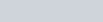$\frac {12.4 A +26}{A+5} = 12.2$
⇒ 12.4A+ 26 = 12.2A + 61
⇒ 0.2A = 61 − 26 = 35
⇒ A = 175

Average MCQ Questions for Competitive Exams

### Finding the Missing Number and Change of Average

Q. 37: Average weight of 25 students of a class is 50 kg. If the weight of the class teacher is included, the average is increased by 1 kg. The weight of the teacher is :
a) 74 kg
b) 75 kg
c) 76 kg
d) 77 kg

Ans : c) 76 kg
Weight of the teacher = 50 + 26 × 1 = 76 kg

Q.38: The average of five numbers is 7. When three new numbers are included, the average of the eight numbers becomes 8.5. The average of three new numbers is :
a) 9
b) 10.5
c) 11
d) 11.5

Ans : c) 11
Sum of the three new numbers = 8 × 8.5 – 5 × 7 = 68 − 35 = 33
Average = 33/3 =11

### Determining the Average Age : Average Questions for Competitive Exams

Q.38: The average age of a mother and her six children is 12 years, which is reduced by 5 years if the age of the mother is excluded. The age of the mother (in years) is :
a) 40
b) 42
c) 48
d) 50

Ans : b) 42
Mother + 6 children ⇒ 12 × 7 = 84 years
6 children ⇒ 6 × 7 = 42 years
∴ Mother’s age ⇒ 84 − 42 = 42 years

Q.39: The average age of a mother and her six children is 12 years, which is reduced by 5 years if the age of the mother is excluded. The age of the mother (in years) is :
a) 40
b) 42
c) 48
d) 50

Ans : b) 42
Mother + 6 children ⇒ 12 × 7 = 84 years
6 children ⇒ 6 × 7 = 42 years
∴ Mother’s age ⇒ 84 − 42 = 42 years

Q.40: The average age of a husband and his wife was 23 years at the time of their marriage. After five years they have a one year old child. The average age of the family now is
a) 19 years
b) 23 years
c) 29.3 years
d) 28.5 years

Ans : a) 19 years
Total present age of family = (2 × 23 + 2 × 5 + 1) = (46 +10+1) = 57
Average = 57/3 = 19

Q.41: Three years ago the average age of a family of 5 members was 17 years. A baby having been born, the average age of the family remains the same today. The age of the baby today is :
a) 1 year
b) 2 years
c) 3 years
d) 1.5 years

Ans : b) 2 years
Total age of 5 members 3 years ago = 17 × 5 = 85 years
Their total present age = 85 + 3 × 5 = 100 years
Total present age of 6 members = 17 × 6 = 102 years
Present age of child  = 102 − 100 = 2 years

Q.42: A person travels by car from city A to city B at an average speed of 63 Km/h and returns at an average speed of 42 Km/h. His average speed for the whole journey?
a) 52.5 Km/h
b) 55.4 Km/h
c) 48.5 Km/h
d) 50.4 Km/h

If the distance covered is the same, then the average speed=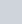$\frac {2xy}{x+y}$
Average Speed =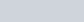$\frac {2*63*42}{63+42}= \frac{252}{5}$ =50.4 Km/h